Function Repository Resource:

# SupportSizeEstimate

Estimate the full size of a set given the number of distinct results in a sample

Contributed by: Ed Pegg Jr
 ResourceFunction["SupportSizeEstimate"][samples,distincts] estimates the full population using a given number of distincts in the samples.

## Examples

### Basic Examples (2)

Ask five hundred people when their birthday is and count the number of distinct results:

 In:=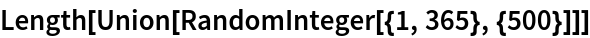Out=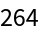Based on that result, make an estimate for the number of days in a year:

 In:=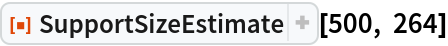Out=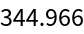Calculate the number of birthdays for Saturn, but keep the number secret:

 In:=Count the number of distinct results in fifty thousand random birthdays on Saturn:

 In:=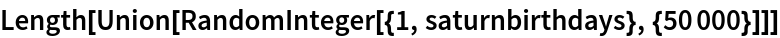Out=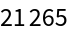With sample sizes 50,000 and 21,265, distinct results estimate how many days per year there are on Saturn:

 In:=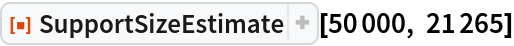Out=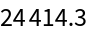### Applications (2)

Sample sorted subsets and use that to estimate the the full support size:

 In:=Out=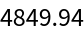In:=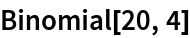Out=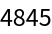### Possible Issues (2)

Sample sorted 4-tuples and use that to estimate the the full support size:

 In:=Out=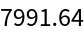This sampling method is not uniformly distributed, so the support size estimate is an undercount:

 In:=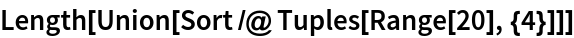Out=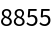If the number of distinct items is the same as the sample size, you will need a larger sample.

## Version History

• 1.0.0 – 11 November 2019